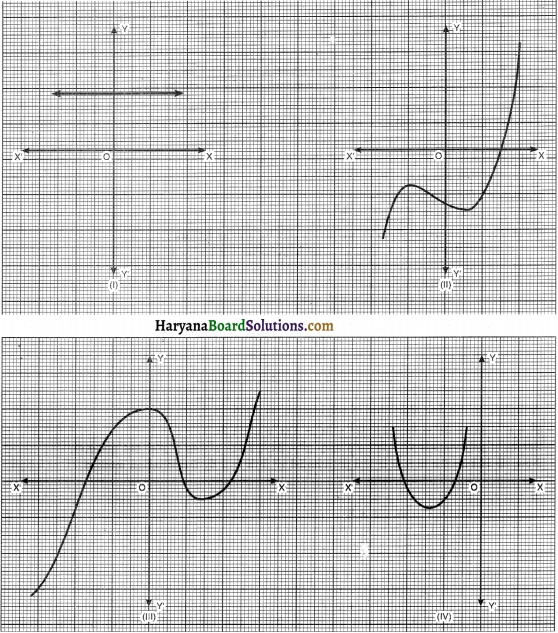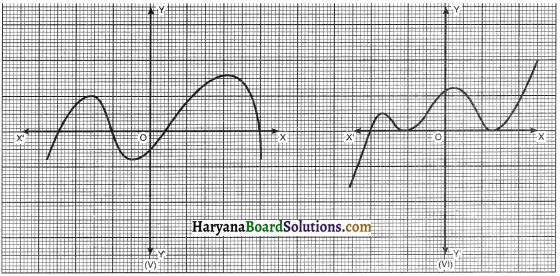# HBSE 10th Class Maths Solutions Chapter 2 Polynomials Ex 2.1

Haryana State Board HBSE 10th Class Maths Solutions Chapter 2 Polynomials Ex 2.1 Textbook Exercise Questions and Answers.

## Haryana Board 10th Class Maths Solutions Chapter 2 Polynomials Exercise 2.1

Question 1.
The graphs of y = p(x) are given in the figure (A) as shown below, for some polynomials p(x). Find the number of zeroes of p(x), in each case.Solution :
(i) The graph is parallel to x-axis. It does not intersect the x-axis. So the number of zeroes is 0.
(ii) The graph intersects the x-axis at one point. So, the number of zeroes is 1.
(iii) The graph intersects the x-axis at three points. So, the number of zeroes is 3.
(iv) The graph intersects the x-axis at two points. So, the number of zeroes is 2.
(v) The graph intersects the x-axis at four points. So, the number of zeroes is 4.
(vi) The graph intersects the x-axis at three points. So, the number of zeroes is 3.